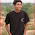### Expected Value

Roll a die, and you get paid what the dice shows. if you want, but you don't have to, you can roll the die again and get paid what the second roll shows instead of the first. What is the expected value?

Update (11/12/09): Solution by Pintu Kumar (CSE, IITB) in comments!!
Update (21/01/10): Solution posted by Pintu was wrong. Correct solution posted in comments by me!!

1.4
Roll it once .. if the value is more than or equals to 3, value of the first roll in counted else we roll again.
So expected value ..
4.5*4/6 + 2/6*3 = 4
Also .. it doesnt make a difference if we take the values strictly greater than 3 on the first roll

2.Nice and elegant solution..

3.I don't how I got this wrong, but this is not the correct solution.

The strategy is roll it once. If the value is more than 3.5, value of the roll in first roll is counted. Else we roll again.

So, expected value = 1/6*(6+5+4) + 1/2(3.5) = 4.25

In Pintu's solution, he miscalculated the expected value of a roll of dice as 3. And hence his solution.

The correct strategy and calculation gives expected value = 4.25 :)